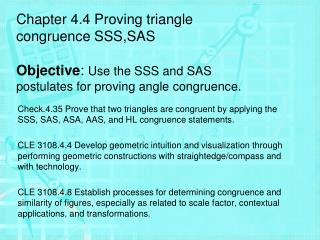DownloadDownload PresentationSide-Side-Side (SSS) Congruence

# Side-Side-Side (SSS) Congruence

Télécharger la présentation## Side-Side-Side (SSS) Congruence

- - - - - - - - - - - - - - - - - - - - - - - - - - - E N D - - - - - - - - - - - - - - - - - - - - - - - - - - -
##### Presentation Transcript

1. Chapter 4.4 Proving triangle congruence SSS,SASObjective: Use the SSS and SAS postulates for proving angle congruence. Check.4.35 Prove that two triangles are congruent by applying the SSS, SAS, ASA, AAS, and HL congruence statements. CLE 3108.4.4 Develop geometric intuition and visualization through performing geometric constructions with straightedge/compass and with technology. CLE 3108.4.8 Establish processes for determining congruence and similarity of figures, especially as related to scale factor, contextual applications, and transformations.

2. Forget past mistakes. Forget failures. Forget everything except what you're going to do now and do it." William Durant Side-Side-Side (SSS) Congruence • If the sides of one triangle are congruent to the sides of a second triangle then the triangles are congruent. • ABC FDE Side-Angle-Side (SAS) Congruence • If two sides and the included angle of one triangle are congruent to two sides and the included angle of another triangle, then the triangles are congruent • ABC FDE F F A D A D E E C B B C

3. Constructions – Congruent Triangles Using Sides Y • Draw a triangle, label the vertices X, Y, and Z • Elsewhere on the paper, use a straight edge to construct segment RS Such that RS  XZ • Using R as the center, draw and arc with radius equal to XY • Using S as the center draw and arc with a radius equal to YZ. • Let T be the point of intersection of the two arcs. • Draw RT and ST to form RST Z X T R S

4. Constructions – Congruent Triangles using 2 sides and an included angle C A • Draw a triangle, label the vertices A, B, and C • Elsewhere on the paper, use a straight edge to construct segment KL Such that KL  BC • Construct and angle congruent to B using KL as a side of the angle and K as the vertex. • Construct JK such that JK  BA. • Draw JL to complete KJL B J K L

5. Copy the two Triangles using the requested methodology B E Using Sides create STV  ABC Using 2 sides and an included angle create XYZ  DEF D A C F

6. Can you prove Congruence? Side, Side, Side - SSS

7. Can you prove Congruence? Side, Angle, Side - SAS

8. Can you prove Congruence? Not enough Information

9. Can you prove Congruence? Not enough Information

10. Can you prove Congruence? Either SSS or SAS

11. B C 4 \$ 3 Page 197 34. 2 Given: ABCD, ADCB, ADDC, ABBC, AD|| BC, AB|| CD 1 A D Prove: ACD CAB Statement • ABCD, ADCB • AC  CA • ADDC, ABBC • AD|| BC, AB|| CD • D and B is a right Angle • 1  4, 2  3 • D B • ACD CAB Reason • Given • Transitive Property • Given • Given • Definition of  lines • Alternate Interior ’s are  • Definition of Right Angles • Def of congruent triangles

12. Y C B Given: ABAC,BYCY Prove: BYA CYA A Statement Reason Given Reflexive Property SSS • ABAC,BYCY • AY  AY • BYA CYA

13. A D Given: X is the midpoint of BD, X is the midpoint of ACProve: DXC BXA X Statement Reason Given Definition of Midpoint Vertical Angles are  SAS B C • X is the midpoint of BD, X is the midpoint of AC • DX  XB, CX  XA • DXC  BXA • DXC BXA

14. Practice Assignment • Block Page 267, 10 – 18 even • Honors; Page 267, 10 – 28 even